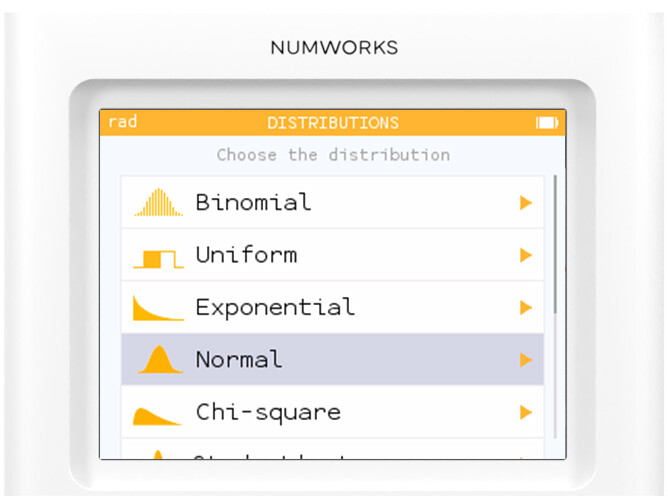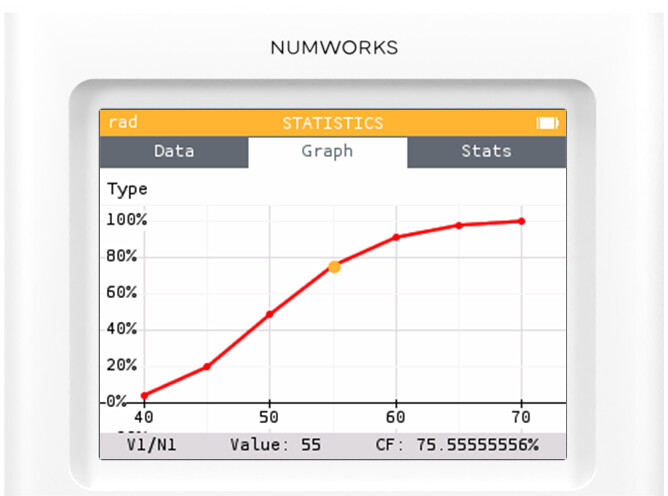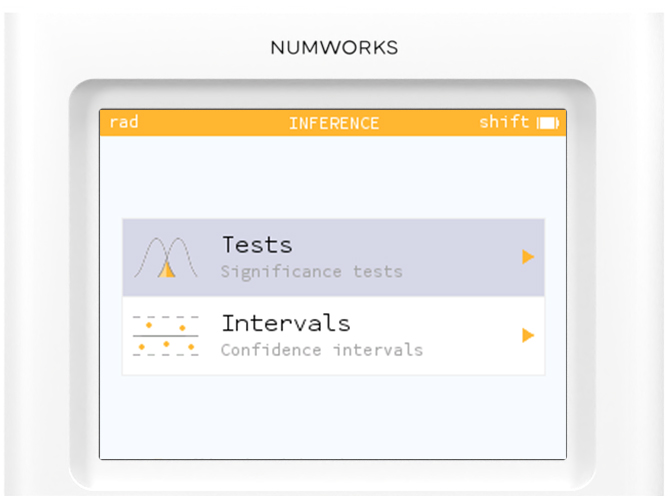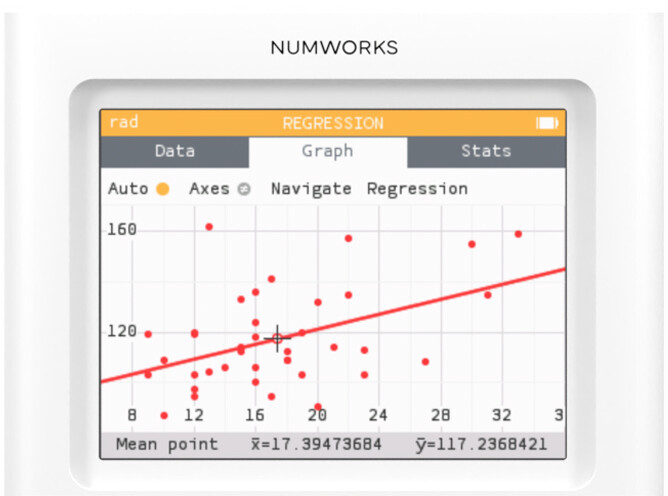# Applications

Navigate easily with our app-based menu

## Calculation

Make math accessible to all!

See mathematical notation exactly like on your paper with results displayed in exact and decimal form.

## Grapher

Obtain beautiful graphs in the perfect viewing window!

While others stop at functions, the NumWorks calculator graphs even more. Easily trace graphs and calculate integrals and derivatives directly on the graph.

## Equations

Solve equations and systems!

Select a template or start from scratch. Your equations will be solved with results given in exact and decimal form.

## For all your statistical needs## Distributions

Compute probabilities from discrete and continuous probability distributions.## Statistics

Enter data, view graphs and analyze summary statistics all in one app.## Inference

Construct confidence intervals and perform significance tests with interactive visuals.## Regression

Create scatterplots and select a regression model that best fits your data.

## Sequences

Forget about complicated syntax. Our template system lets you write recursive and explicit sequences with ease. You can even link your sequences together!

## Finance

Solve financial problems involving simple or compound interest with ease.

## Elements

Our interactive periodic table of elements provides everything you need to know about each element!